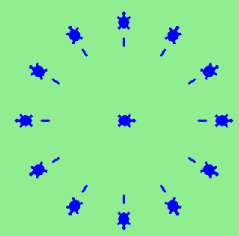# 5.10. Exercises¶

1. Write a program that prints We like Python's turtles! 100 times.

1. Turtle objects have methods and attributes. For example, a turtle has a position and when you move the turtle forward, the position changes. Think about the other methods shown in the Summary of Turtle Methods page. Which attibutes, if any, does each method relate to? Does the method change the attribute?

1. Use for loops to make a turtle draw these regular polygons (regular means all sides the same lengths, all angles the same):

• An equilateral triangle

• A square

• A hexagon (six sides)

• An octagon (eight sides)

# draw an equilateral triangle
import turtle

wn = turtle.Screen()
norvig = turtle.Turtle()

for i in range(3):
norvig.forward(100)

# the angle of each vertice of a regular polygon
# is 360 divided by the number of sides
norvig.left(360/3)

wn.exitonclick()

# draw a square
import turtle

wn = turtle.Screen()
kurzweil = turtle.Turtle()

for i in range(4):
kurzweil.forward(100)
kurzweil.left(360/4)

wn.exitonclick()

# draw a hexagon
import turtle

wn = turtle.Screen()
dijkstra = turtle.Turtle()

for i in range(6):
dijkstra.forward(100)
dijkstra.left(360/6)

wn.exitonclick()

# draw an octogon
import turtle

wn = turtle.Screen()
knuth = turtle.Turtle()

for i in range(8):
knuth.forward(75)
knuth.left(360/8)

wn.exitonclick()

1. Write a program to draw a shape like this: